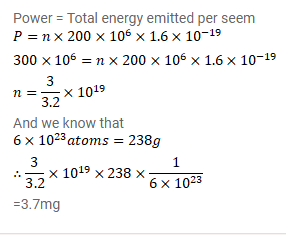# A uranium reactor develops thermal energy at a rate of

Question:

A uranium reactor develops thermal energy at a rate of $300 \mathrm{MW}$. Calculate the amount of $235 \mathrm{U}$ being consumed every second. Average energy released per fission is $200 \mathrm{MeV}$.

Solution: